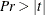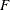# The VARMAX Procedure

### Printed Output

The default printed output produced by the VARMAX procedure is described in the following list:

• descriptive statistics, which include the number of observations used, the names of the variables, their means and standard deviations (STD), their minimums and maximums, the differencing operations used, and the labels of the variables

• a type of model to fit the data and an estimation method

• a table of parameter estimates that shows the following for each parameter: the variable name for the left-hand side of equation, the parameter name, the parameter estimate, the approximate standard error, t value, the approximate probability (), and the variable name for the right-hand side of equations in terms of each parameter

• the innovation covariance matrix

• the information criteria

If PRINT=ESTIMATES is specified, the VARMAX procedure prints the following list with the default printed output:

• the estimates of the constant vector (or seasonal constant matrix), the trend vector, the coefficient matrices of the distributed lags, the AR coefficient matrices, and the MA coefficient matrices

• the ALPHA and BETA parameter estimates for the error correction model

• the schematic representation of parameter estimates

If PRINT=DIAGNOSE is specified, the VARMAX procedure prints the following list with the default printed output:

• the cross-covariance and cross-correlation matrices of the residuals

• the tables of test statistics for the hypothesis that the residuals of the model are white noise:

• Durbin-Watson (DW) statistics

•test for autoregressive conditional heteroscedastic (ARCH) disturbances

•test for AR disturbance

• Jarque-Bera normality test

• Portmanteau test